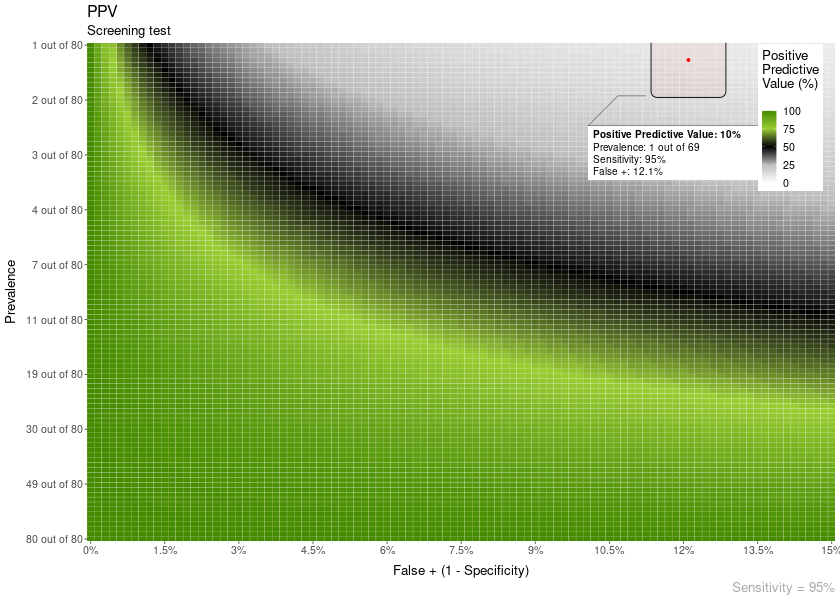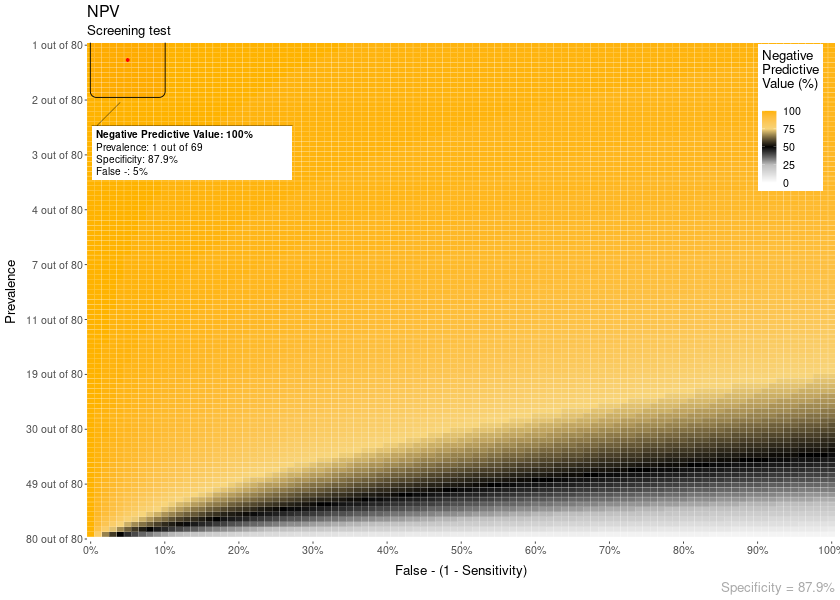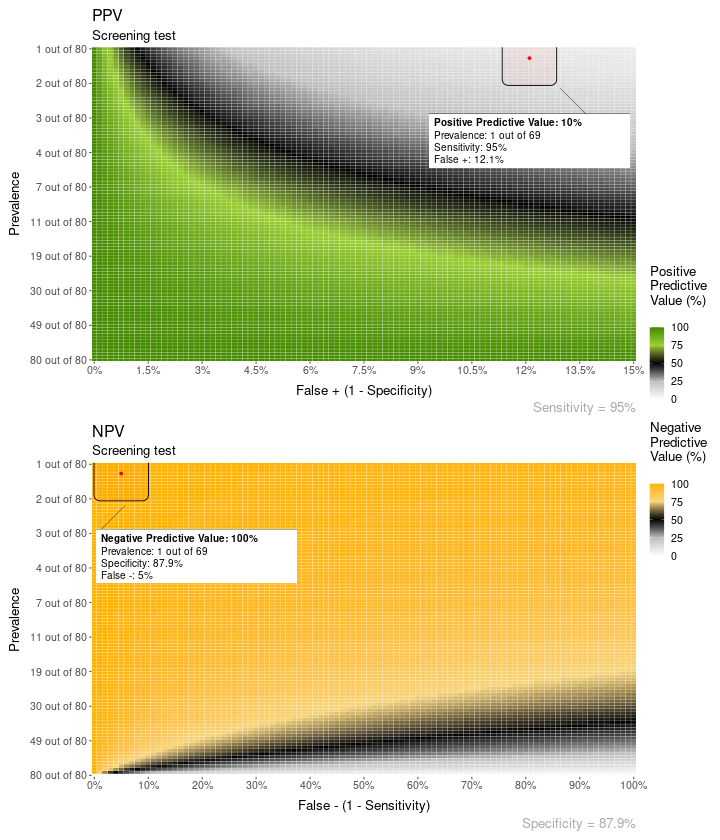# Screening tests and PPV vs NPV

#### 2022-01-03

Screening tests are applied to asymptomatic people with the hope to catch a disease in the early stages.

Screening tests are by definition applied to populations where the prevalence of the condition is low (most people are healthy). This simple fact has consequences for how much we can trust their + and - results, the Positive Predictive Value (PPV) and Negative Predictive Value (NPV) of the test, respectively.

Please, keep in mind the goal of this vignette is to exemplify how to use the BayesianReasoning package. None of the information contained here should be taken as medical advice.

## PPV and NPV definitions

PPV, formally $$P(Disease \mid +)$$ is the probability of having a disease given a test result is +.

$$P(Disease \mid +) = \frac{TruePositives}{TruePositives + FalsePositives}$$

NPV, formally $$P(Healthy \mid -)$$ is the probability of being healthy given a test result is -.

$$P(Healthy \mid -) = \frac{TrueNegatives}{TrueNegatives + FalseNegatives}$$

## Example

We will use as an example Mammography at 50 years old as a screening test to detect Breast Cancer.

### PPV

The PPV of Mammography at 50 years old in the general population is relatively low.


PPV_plot = PPV_heatmap(
min_Prevalence = 1, max_Prevalence = 80,
Sensitivity = 95,
limits_Specificity = c(85, 100),
overlay = "area",
overlay_prevalence_1 = 1,
overlay_prevalence_2 = 69,
overlay_position_FP = 12.1,
label_title = "PPV",
label_subtitle = "Screening test"
)### NPV

The NPV of Mammography at 50 years old in the general population is very high.


NPV_plot = PPV_heatmap(
PPV_NPV = "NPV",
min_Prevalence = 1,
max_Prevalence = 80,
Specificity = 87.9,
overlay = "area",
overlay_prevalence_1 = 1,
overlay_prevalence_2 = 69,
overlay_position_FN = 5,
label_title = "NPV",
label_subtitle = "Screening test"
)
#> Warning in process_variables(min_Prevalence = min_Prevalence, max_Prevalence
#> = max_Prevalence, : * Sensitivity and limits_Specificity are NULL. Setting
#> Sensitivity = 95 and limits_Sensitivity = c(90, 100)
#> Warning in process_variables(min_Prevalence = min_Prevalence, max_Prevalence = max_Prevalence, :
#> [WARNING]: overlay_position_FN (5) is < min_FN (100)
#> [EXPECTED]: overlay_position_FN should be <= min_FN.
#> [CHANGED]: min_FN = 0### Combined PPV + NPV

Combining both PPV and NPV shows how negative results of Mammography at 50 years old in the general population are very trustworthy, but positive results are not.

We can plot the PPV and NPV plots side by side using {patchwork}:


(PPV_plot / NPV_plot) +  plot_layout(guides = 'collect')## Sources

Breast Cancer screening information:

Theoretical overview of the technical concepts:

Practical explanation about the importance of understanding PPV: